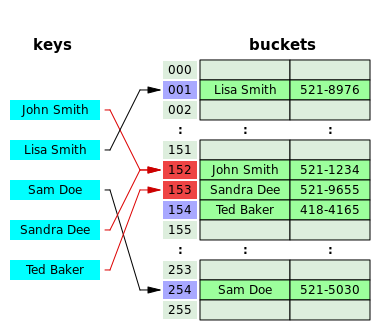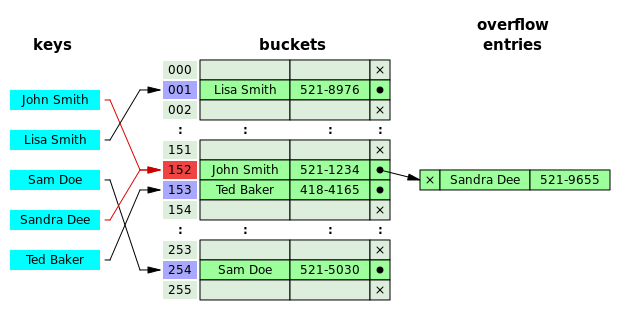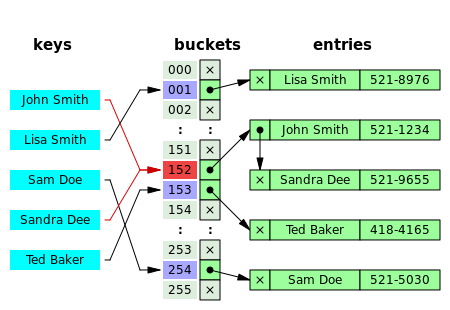# 数据结构——哈希表

2019-01-05

## 1 构造散列函数

1. 直接定址法： 取关键字或关键字的某个线性函数值为散列地址。即 $hash(k)=k$$hash(k)=a\cdot k+b$，其中 $a,b$为常数（这种散列函数叫做自身函数）;
2. 除留余数法： 取关键字被某个不大于散列表表长$m$的数$p$除后所得的余数为散列地址。即$hash(k)=k\,{\bmod \,}p,p\leq m$。不仅可以对关键字直接取模，也可在折叠法、平方取中法等运算之后取模。对$p$的选择很重要，一般取素数或$m$，若$p$选择不好，容易产生冲突。
3. 数字分析法： 假设关键字是以$r$为基的数，并且哈希表中可能出现的关键字都是事先知道的，则可取关键字的若干数位组成哈希地址。比如：比如有一组value1=112233，value2=112633，value3=119033，针对这样的数我们分析数中间两个数比较波动，其他数不变。那么我们取key的值就可以是key1=22,key2=26,key3=90。
4. 折叠法： 将关键字分割成位数相同的几部分（最后一部分的位数可以不同），然后取这几部分的叠加和（舍去进位）作为哈希地址。比如value=135790，要求key是2位数的散列值。那么我们将value变为13+57+90=160，然后去掉高位“1”,此时key=60，哈哈，这就是他们的哈希关系，这样做的目的就是key与每一位value都相关，来做到“散列地址”尽可能分散的目地。
5. 随机数法
6. 平方取中法

## 2 处理冲突

### 2.1 开放定址法### 2.2 拉链法